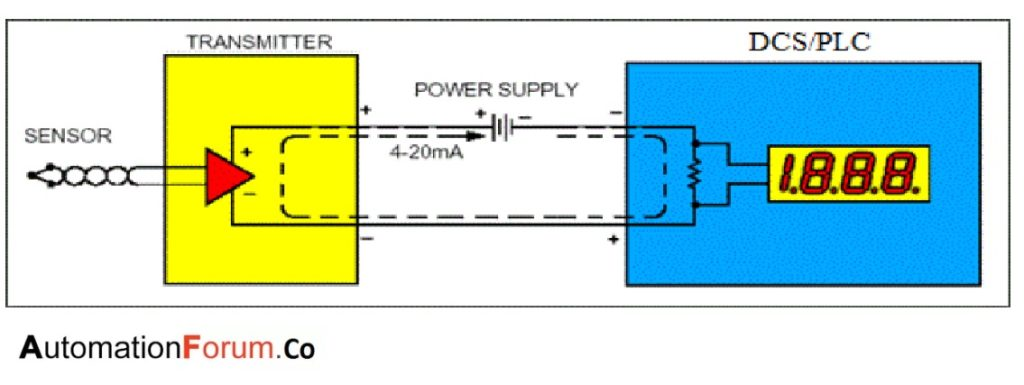# What is a 4-20 mA Current Loop?## Introduction

Before the age of electronic circuitry the whole process is controlled by mechanically.Facilities used pneumatic control signals where controllers were powered by varying pressures of compressed air.The 3 -15psi standard became the standard.Easier to differentiate a live zero (3 psi) signal from a failure in the system (0 psi).In the 1950s, as electronic systems became less expensive, current input became the preferred and more efficient process control signal. The 4-20 mA range later became the standard for similar reasons as 3-15 psi did.

## What is a 4-20 mA Current Loop?

Consider the simple DC circuit below, consisting of a power supply and three loads. with the voltage of the supply labeled as Vtotal.Current then flows through the loop, passing through each load. The voltage drop at each load can be calculated from Ohm’s Law. The voltage drop V1 across R1 is:

V1 = I x R1

Every element in the loop either provides voltage or has a voltage drop.

However, the current, I is the same everywhere in the loop. This is the critical principle of the 4-20 mA loop. Current is the same in all places throughout the loop. This is why using current as a means of conveying process information is so reliable.

## Why Use a Current Loop?

The 4-20mA current loop shown in Figure  is a common method of transmitting sensor information in many industrial process-monitoring applications. A sensor is a device used to measure physical parameters such as temperature, pressure, speed, liquid flow rates, etc.Transmitting sensor information via a current loop is particularly useful when the information has to be sent to a remote location over long distances (1000 feet, or more). The loop’s operation is straightforward: a sensor’s output voltage is first converted to a proportional current, with 4mA normally representing the sensor’s zero-level output, and 20mA representing the sensor’s full-scale output. Then, a receiver at the remote end converts the 4-20mA current back into a voltage which in turn can be further processed by a computer or display module.

## Advantages of 4 -20 ma signals

The 4-20 mA current loop is the dominant standard in many industries.

• It is the simple
• reduce initial setup cost
• It uses less wiring and connections than other signals
• signal will not loss during long distance transmissions like voltages
• It is less sensitive to background electrical noise.
• Since 4 mA is equal to 0% output, it is incredibly simple to detect a fault in the system.

## Disadvantages of 4 -20 ma signals

• Current loops can only transmit one particular process signal.
• Multiple loops must be created in situations where there are numerous process variables that require transmission.
• Running so much wire could lead to problems with ground loops if independent loops are not properly isolated. These isolation requirements become exponentially more complicated as the number of loops increases.
Scroll to Top